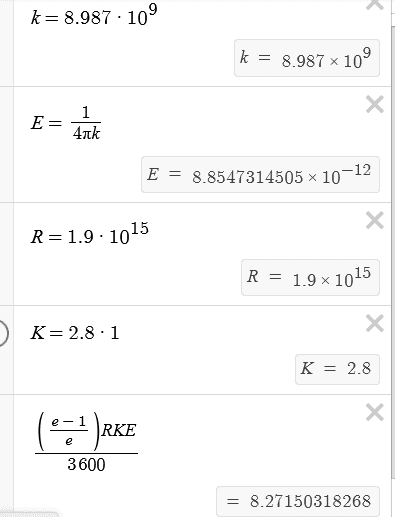# Current leakage between the charged plates of a capacitor

• archaic

#### archaic

Homework Statement
A charged plate capacitor is filled with a dielectric of dielectric constant ##\kappa=2.50## and resistivity ##\rho=1.90\times10^{15}\,\Omega m##. It is first charged to ##Q=8.00\,\mu C##.
1) What is the magnitude of the leakage current in amps?
2) What is time interval (in hours) over which the charge on the capacitor will fall to ##1/e## of its initial value?
Relevant Equations
$$I=\frac{A\Delta V}{\ell\rho}$$$$C=\frac{\kappa\epsilon_0A}{\ell}$$
1)$$\frac{Q}{\Delta V}=\frac{\kappa\epsilon_0A}{\ell}\Leftrightarrow\Delta V=\frac{Q\ell}{\kappa\epsilon_0A}$$$$I=\frac{A\Delta V}{\ell\rho}=\frac{Q}{\kappa\epsilon_0\rho}$$
2) The charge is decreasing by ##\Delta Q##, so ##Q(t)=Q-\Delta Q##.$$I=\frac{\Delta Q}{\Delta t}\Leftrightarrow\Delta Q=\frac{Q}{\kappa\epsilon_0\rho}\Delta t$$$$Q-\Delta Q=\frac{Q}{e}\Leftrightarrow\frac{Q}{\kappa\epsilon_0\rho}\Delta t=\frac{e-1}{e}Q\Leftrightarrow\Delta t=\left(\frac{e-1}{e}\right)\kappa\epsilon_0\rho$$
I divide by ##3600## to get the result in hours.

I am getting that 2) is wrong, though. I entered the values correctly. Here's the calculation as I have done it on desmosIs ##I=\Delta Q/\Delta t## a wrong assumption in this case?

I think you could try take the limit in $$\Delta Q = \frac{Q}{\kappa \rho \varepsilon }\Delta t$$, not only, i think we need a negative sign here, otherwise
$$\Delta Q$$
would end being positive.

I think you could try take the limit in $$\Delta Q = \frac{Q}{\kappa \rho \varepsilon }\Delta t$$
Hm$$\frac{dQ}{dt}=-\frac{Q}{\kappa\epsilon_0\rho}\Leftrightarrow-\kappa\epsilon_0\rho\frac{dQ}{Q}=dt\Leftrightarrow-\kappa\epsilon_0\rho\ln\left(\frac{Q'}{Q_0}\right)=\Delta t$$with ##Q'=Q_0/e##, I get ##\Delta t=\kappa\epsilon_0\rho##.
i think we need a negative sign here
yep, I added that in ##Q-\Delta Q##, but not in the first formula since the question asked for the magnitude.
This is it! Thank you. :)